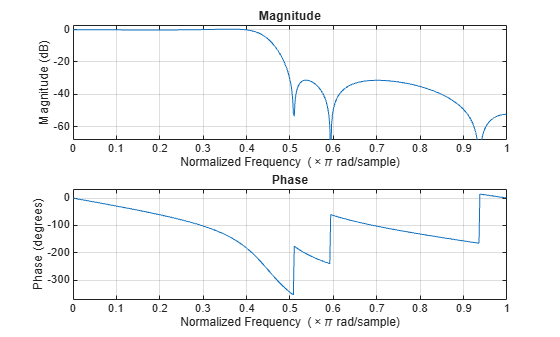# iirlpnormc

Constrained least Pth-norm optimal IIR filter

## Syntax

```[num,den] = iirlpnormc(n,d,f,edges,a) [num,den] = iirlpnormc(n,d,f,edges,a,w) [num,den] = iirlpnormc(n,d,f,edges,a,w,radius) [num,den] = iirlpnormc(n,d,f,edges,a,w,radius,p) [num,den] = iirlpnormc(n,d,f,edges,a,w,radius,p,dens) [num,den] = iirlpnormc(n,d,f,edges,a,w,radius,p,dens,initnum,initden) [num,den,err] = iirlpnormc(...) [num,den,err,sos,g] = iirlpnormc(...) ```

## Description

`[num,den] = iirlpnormc(n,d,f,edges,a)` returns a filter having numerator order `n` and denominator order `d` which is the best approximation to the desired frequency response described by `f` and `a` in the least-pth sense. The vector `edges` specifies the band-edge frequencies for multi-band designs. A constrained Newton-type algorithm is employed. `n` and `d` should be chosen so that the zeros and poles are used effectively. See the Hints section. Always check the resulting filter using `fvtool`.

`[num,den] = iirlpnormc(n,d,f,edges,a,w)` uses the weights in `w` to weight the error. `w` has one entry per frequency point (the same length as `f` and `a`) which tells `iirlpnormc` how much emphasis to put on minimizing the error in the vicinity of each frequency point relative to the other points. `f` and `a` must have the same number of elements, which can exceed the number of elements in `edges`. This allows for the specification of filters having any gain contour within each band. The frequencies specified in `edges` must also appear in the vector `f`. For example,

```[num,den] = iirlpnormc(5,5,[0 .15 .4 .5 1],[0 .4 .5 1],... [1 1.6 1 0 0],[1 1 1 10 10])```

designs a lowpass filter with a peak of 1.6 within the passband.

`[num,den] = iirlpnormc(n,d,f,edges,a,w,radius)` returns a filter having a maximum pole radius of `radius` where 0<`radius`<1. `radius` defaults to 0.999999. Filters that have a reduced pole radius may retain better transfer function accuracy after you quantize them.

`[num,den] = iirlpnormc(n,d,f,edges,a,w,radius,p)` where `p` is a two-element vector [`pmin pmax`] allows for the specification of the minimum and maximum values of `p` used in the least-pth algorithm. Default is [2 128] which essentially yields the L-infinity, or Chebyshev, norm. `pmin` and `pmax` should be even. If `p` is `'inspect'`, no optimization will occur. This can be used to inspect the initial pole/zero placement.

`[num,den] = iirlpnormc(n,d,f,edges,a,w,radius,p,dens)` specifies the grid density `dens` used in the optimization. The number of grid points is `(dens*(n+d+1))`. The default is 20. `dens` can be specified as a single-element cell array. The grid is not equally spaced.

`[num,den] = iirlpnormc(n,d,f,edges,a,w,radius,p,dens,initnum,initden)` allows for the specification of the initial estimate of the filter numerator and denominator coefficients in vectors `initnum` and `initden`. This may be useful for difficult optimization problems. The pole-zero editor in Signal Processing Toolbox™ software can be used for generating `initnum` and `initden`.

`[num,den,err] = iirlpnormc(...)` returns the least-Pth approximation error `err`.

`[num,den,err,sos,g] = iirlpnormc(...)` returns the second-order section representation in the matrix SOS and gain G. For numerical reasons you may find SOS and G beneficial in some cases.

## Hints

• This is a weighted least-pth optimization.

• Check the radii and location of the resulting poles and zeros.

• If the zeros are all on the unit circle and the poles are well inside of the unit circle, try increasing the order of the numerator or reducing the error weighting in the stopband.

• Similarly, if several poles have a large radius and the zeros are well inside of the unit circle, try increasing the order of the denominator or reducing the error weight in the passband.

• If you reduce the pole radius, you might need to increase the order of the denominator.

The message

`Poorly conditioned matrix. See the "help" file.`

indicates that `iirlpnormc` cannot accurately compute the optimization because either:

1. The approximation error is extremely small (try reducing the number of poles or zeros — refer to the hints above).

2. The filter specifications have huge variation, such as `a=[1 1e9 0 0]`.

## Examples

collapse all

This example returns a lowpass filter whose pole radius is constrained to 0.8.

```[b,a,err,s,g] = iirlpnormc(6,6,[0 .4 .5 1],[0 .4 .5 1],... [1 1 0 0],[1 1 1 1],.8); fvtool(b,a);```The magnitude response shows the lowpass nature of the filter. The pole/zero plot following shows that the poles are constrained to 0.8 as specified in the command.

`fvtool(b,a,'polezero');`Antoniou, A., Digital Filters: Analysis, Design, and Applications, Second Edition, McGraw-Hill, Inc. 1993.

## SupportGet trial now